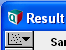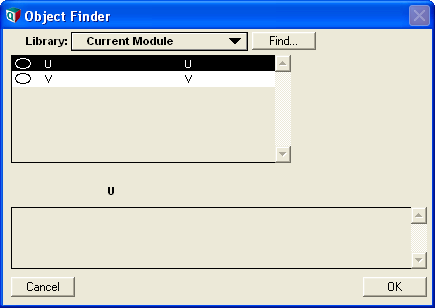# XY comparison

Normally, a graph plots the values of a variable V along the vertical (Y) axis against one of its indexes along the horizontal (X) axis.

With XY comparison, you can plot the values of a variable V along the vertical (Y) axis against the values of another variable along the horizontal (X) axis.

## Use XY Comparison to create a scatter plot

For example, if you graph the sample view of an uncertain variable V, it normally plots the values of V on the vertical axis and the Run index (1 to SampleSize) along the horizontal axis. In a scatter plot, you can plot the values of V on the vertical (Y) axis against another uncertain variable, U, along the horizontal (X) axis. Each point on the plot gets its two values from U and V, respectively. In this case, Run is the Common index, being common to both U and V arrays.

Suppose V and U are uncertain and dependent variables:

Variable V := Normal(5, 2)
Variable U := V + Uniform(-1, 3)

Here is how to use XY comparison to create a scatter plot of V against U:

1. Display the graph for uncertain quantity V.2. Select the Sample view.3. Make sure you are in Edit mode, so that the XY button is displayed in top right of the Graph window.

4. Click the XY button to open the XY Comparison sources dialog.

5. Select checkbox Use another variable [Compare against these variables with a common index:]6. Click Add… to open the Object Finder dialog

7. In the Object Finder dialog, select variable U, and click OK.

8. U is now in the Use another variable list in the XY Comparison sources. [Note that the common index is set to Run.] Click OK.9. The graph now shows the scatter plot of V on vertical (Y) axis and U on the horizontal (X) axis. Each point is a random sample over index Run (from 1 to 100).## Common index

Each point on the graph represents a value of the common index. The common index should be common to the current variable V and other variables or expressions to which you wish to compare V. If there is more than one such index, it shows the common index as a menu so that you select the one you want. By default, it selects what it considers the most plausible, usually the index with most elements.

## Use comparison index

Check this box if you want to compare one slice of the current variable against another slice, with each slice selected from the Comparison index.

The Comparison index is an index of the variable. After clicking OK to return to graph window, you will be able to select slices from the common index from the Horizontal and Vertical menus to display along those axes.

### Horizontal axis

This menu lets you select which variable to plot on the horizontal (X) axis. It appears in the result window when there is more than one alternative variable to display, for example, if there is more than one variable or expression in the Compare with list – or if you have selected a Comparison index, it lets you select which slice you want to plot.

### Vertical axis

This menu lets you select which variable to plot on the vertical axis. It appears on the result window when you have selected a comparison index from the variable. It lists the slice values from the Comparison index, from which you can choose the one to plot along the vertical (Y) axis.

## XY Comparison sources dialog

You can compare the variable not only with other variables, but also with simple expressions calling a function or operator.

1. Click Add.. to open the Object Finder dialog.
2. Press on the Library menu, and select the library (or module) that contains the function or operator you want.
3. Select the function or operator, and fill in its parameters (or operands)
4. Click OK to add expression to Other variables and expressions.

### Remove

Select one or more variables or expressions, and click Remove to remove them from the list.

### Use Comparison index

You can compare (plot against one another) slices of the current variable over the Comparison index.

## History

Introduced in Analytica 4.0.